tamoshop.com
gDraw for iPhone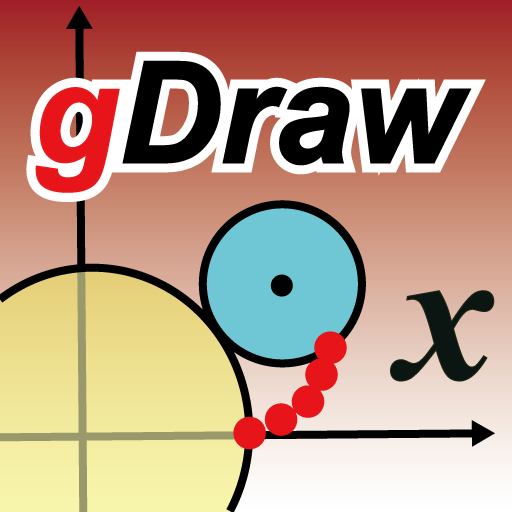Screen shots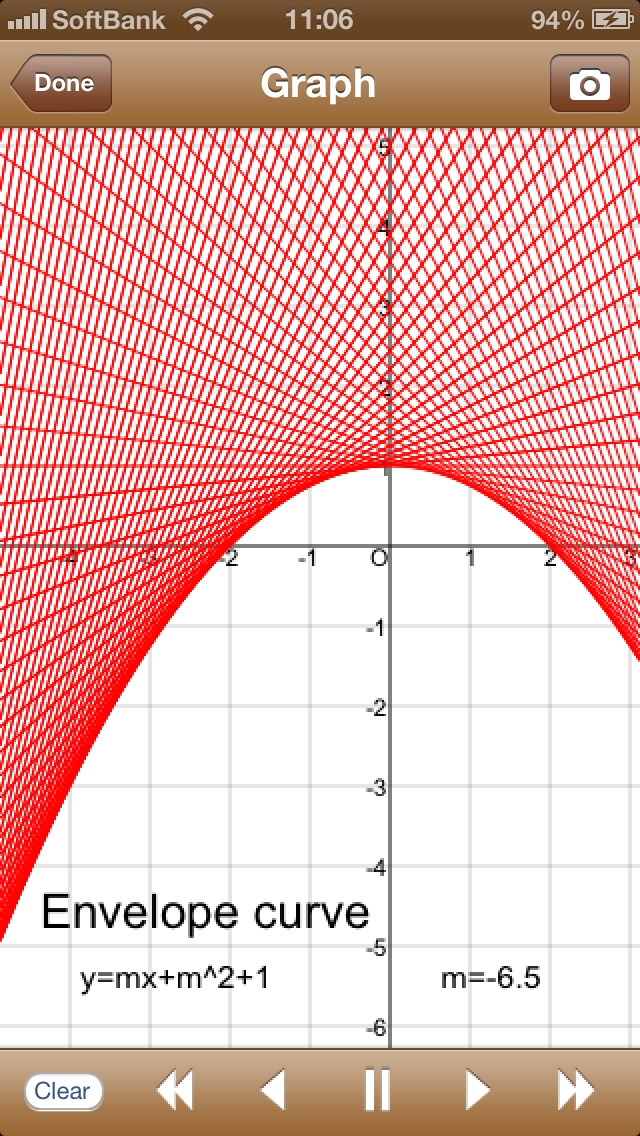�@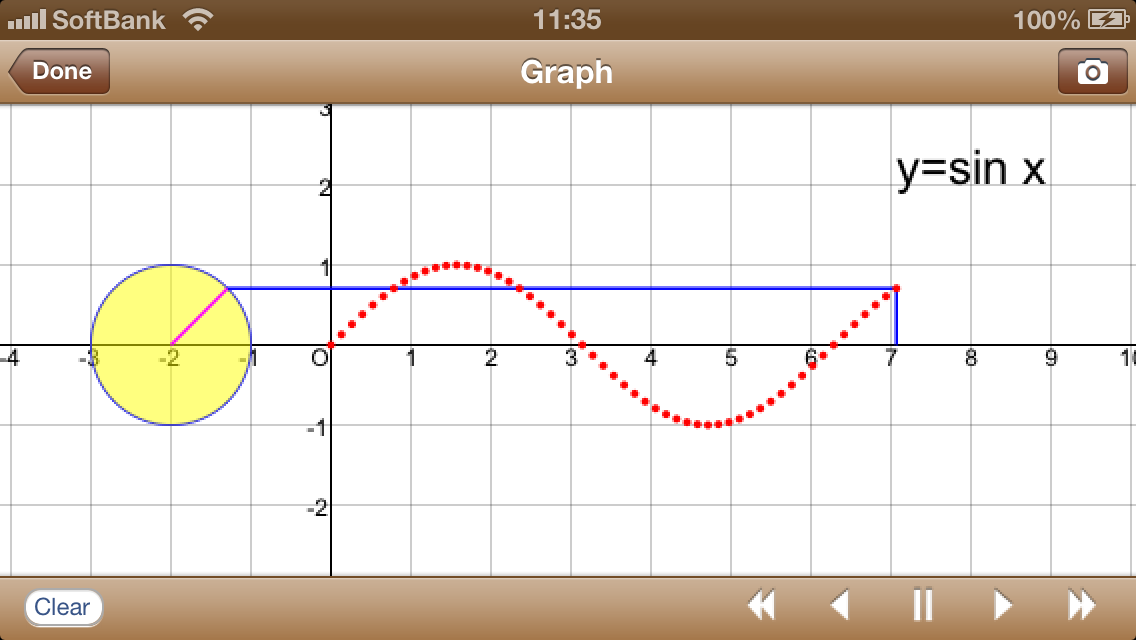�@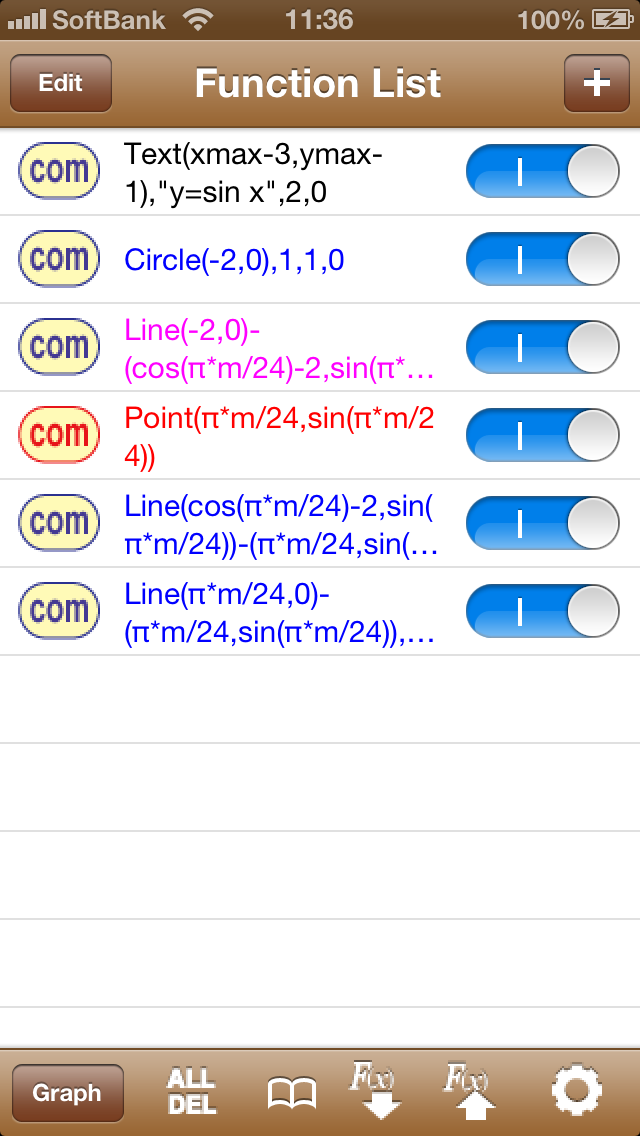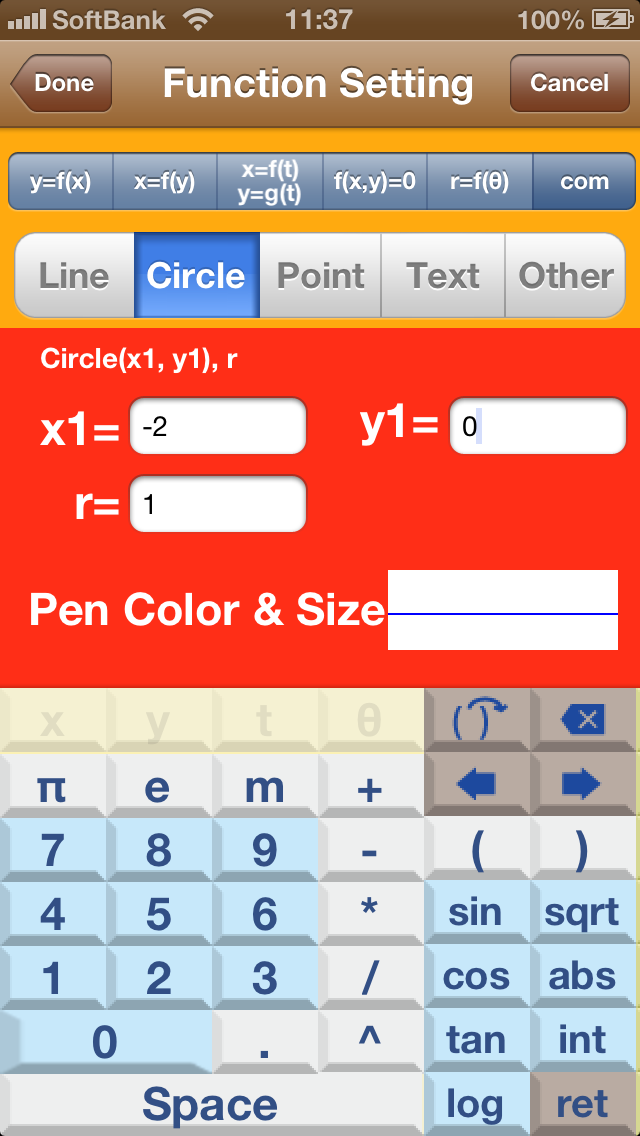�@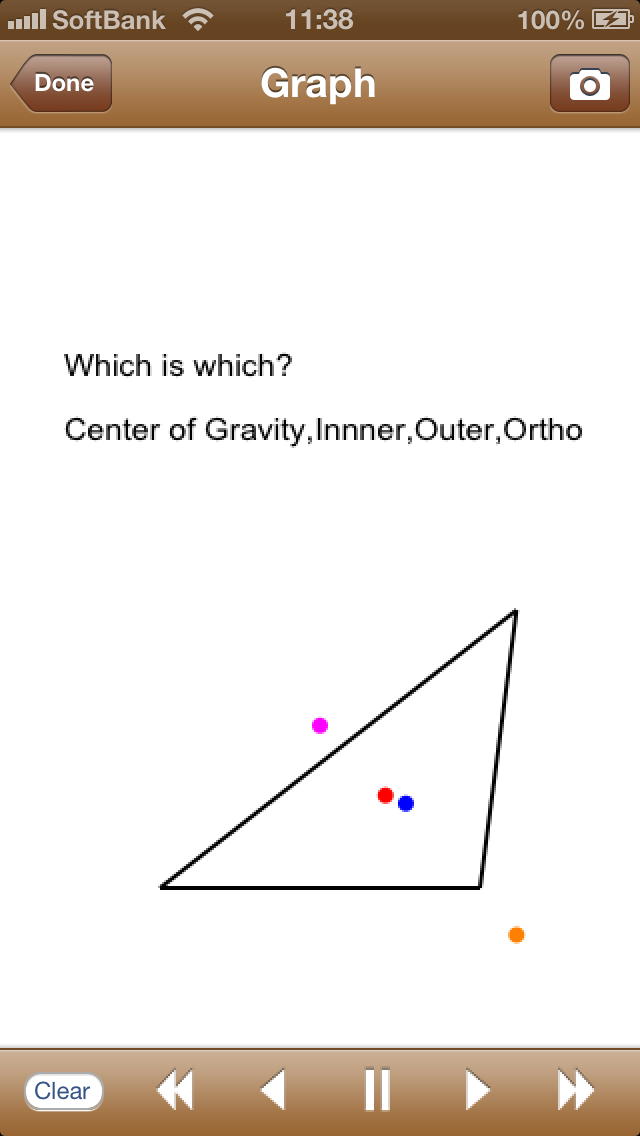What kind of application is gDraw?

Move! Move! It moves! You can draw and move your graphs!
Setting invariables in the formula and changing the value, you can move your graphs freely!

AMAZINGLY MANY KINDS OF GRAPHS!
You can draw many kinds of graphs by setting function such as y=f(x), x=f(y), x=f(t) and y=g(t), f(x,y)=0, pole equation.

MOVE GRAPHS! This is the greatest feature of this app!
We prepared "m" as an invariable.
You can change the value continuously and see the graph moving.
Using this feature, you can see many interesting moves, such as simple oscillation, cycloid curve, synthesis of waves.

ORIGINAL KEYBOARD!
We prepared the original keyboard specialized for mathematical formulas.
You can type mathematical formulas very easily.

SEE MANY GRAPHS SIMULTANEOUSLY!
You can set unlimited number of graphs and see them simultaneously.

VERY CONVENIENT TOOL "com".
By using this, you can draw line segments, circles, points and texts very easily.
For example, you can draw a line segments by setting the starting point and the end point.

MANY INTERESTING SAMPLES!
They are not only interesting, but also help you to master this app.

TAKE A PHOTO OF YOUR GRAPH!
You easily take a photo of your graph by pushing �gphoto button�h.

gDraw Function Lists

tamo practice

tamo practice2

news